# Two bricks

One brick weighs 1kg and half a brick, how many weigh two bricks?

m =  4 kg

### Step-by-step explanation: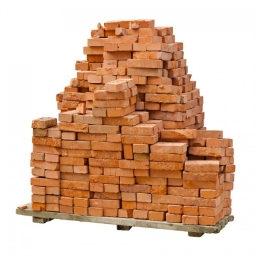Did you find an error or inaccuracy? Feel free to write us. Thank you!Tips to related online calculators
Do you have a linear equation or system of equations and looking for its solution? Or do you have a quadratic equation?

## Related math problems and questions:

• Brick weightThe brick weighs 2 kg and a half bricks. How much does one brick weigh?
• Bricks II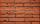3/4 bricks weighs 6 kg and 2/3 bricks. How weighs one whole brick?
• Brick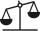Isosceles scale has on one side all brick and second weight 1 kg and 1/4 of brick. The balance is in equilibrium. What is the weight of a brick?
• Can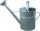Watering can full of water weighs 10 kg. Half-full can weighs 5.5 kg. How much weigh can?
• Children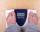Margaret and Zdena weigh the same and Petra 3 kg more. Together weigh 156 kg. How much do they weigh?
• Rails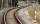18 m railway weighs 1260 kg. How much weighs 100 m of welded railways?
• The devils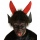The devils weighed in hell with Dorota. They found that Dorota and the two devils weigh 250 kg together, and Dorota and the four devils weigh 426 kg. All the devils weigh the same. How Much Does Dorota Weigh?
• One kilogramThe apple weighs 125 grams and half apple. How many apples weigh 1 kilogram?
• Brick weightHalf the weight of a brick plus 20 pounds is equal to 1/3 the weight of the brick plus 30 pounds. How much does the brick weigh?
• Tess boughtTess bought 6 dressed chickens. Their weights are tagged as follows: 1.4 kg, 1.9 kg, 2.05 kg, 0.94 kg, 1.1 kg and 1.45 kg. What is the total weight of the six chickens
• Brick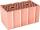Brick weight before drying is 5.3 kg. After drying, the bricks is 1 kg lighter and after firing is 0.7 kg lighter than after drying. What is the weight of the brick after drying and firing?
• Bag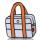Nelly found interesting point. An empty bag weighs 4 kg less than full. And empty bag is 5 times easier than full. How many kg weigh things in this bag?
• BucketThe bucket half filled with water weighs 5.55 kg, the full bucket weighs 9.85 kg. How much does the bucket weigh?
• HomeworkMother and Kate together weigh 24.5 kg more than the father. Father weighs 7.6 kg more than mother. Father weight 44.8 kg more than Kate. Determine weights of father mother and Kate.
• Weigh in totalI put 3/5 kg of grapes into a box which is 1/4kg in weight. How many kilograms do the grapes and the box weigh in total?
• Volunteers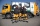Volunteers helped clean up 8.2 kg of trash in one neighborhood and 11 1/2 kg in another. They sent 2 3/5 kg to be recycled and threw the rest away. How many kilograms of trash did they throw away?
• Freight train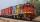Twenty-five identical freight wagons are connected behind the locomotive. The weight of the locomotive and all wagons is 1009000 kg. The locomotive weighs 84 tons. How many kilograms does one freight car weigh?